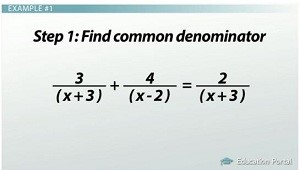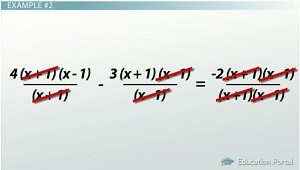# How to Solve a Rational Equation

#### Video Lesson on How to Solve a Rational Equation

This lesson video Not available at this time available video coming soon

### How to Solve a Rational Equation

A rational equation is one that contains fractions. Yes, we will be finding a common denominator that has 'x's. But no worries! Together we will use a process that will help us solve rational equations every time!

### Rational Equations

A rational equation is an equation that contains fractions with xs in the numerator, denominator or both. Here is an example of a rational equation: (4 / (x + 1)) - (3 / (x - 1)) = -2 / (x^2 - 1).

Let's think back for a moment about solving an equation with a fraction. 1/3 x = 8. We think of the 3 in the denominator as being a prisoner, and we want to release it. To set the 3 free, we multiply both sides of the equation by 3. Think of it as 3 letting both sides of the equation know he's leaving. 3 (1/3 x) = 8 (3).

This process freed our denominator and got rid of the fraction - x = 24. It is also the process we use to solve rational equations with one extra step. In rational equations, sometimes our solution may look good, but they carry a virus; that is, they won't work in our equation. These are called extraneous solutions. The steps to solve a rational equation are:

1. Find the common denominator.
2. Multiply everything by the common denominator.
3. Simplify.
4. Check the answer(s) to make sure there isn't an extraneous solution.
5. Let's solve a couple together.

### Example #1

Example number one: solve. Remember to check for extraneous solutions. (3 / (x + 3)) + (4 / (x - 2)) = 2 / (x + 3).

Our first step is to figure out the terms that need to be released from the denominators. I look at 3 / (x + 3). I write down (x + 3) as one of my common denominators. I look at 4 / (x - 2). I write down (x - 2) as another part of my common denominator. I look at 2 / (x + 3). Since I already have (x + 3) written in my denominator, I don't need to duplicate it.

Next, we multiply everything by our common denominator - (x+3)(x-2). This is how that will look: ((3(x + 3)(x - 2)) / (x + 3)) + ((4(x + 3)(x - 2)) / (x - 2)) = (2(x + 3)(x - 2)) / (x + 3))

It isn't easy for the denominators to be released; there is a battle, and like terms in the numerator and denominator get canceled (or slashed). Slash (or cancel) all of the (x + 3)s and (x - 2)s in the denominator and numerator. Our new equation looks like: 3(x - 2) + 4(x + 3) = 2(x - 2).In example #1, the first step is finding the common denominator.

Distribute to simplify: (3x - 6) + (4x + 12) = 2x - 4. Collect like terms and solve. 3x + 4x = 7x, -6 + 12 = 6. We end up with 7x + 6 = 2x - 4.

Subtract 2x from both sides: 7x - 2x = 5x. Subtracting from the other side just cancels out the 2x, and we get 5x + 6 = -4. Subtract 6 from both sides: -4 - 6 = -10. Again, subtracting 6 will cancel out the +6, so we end up with 5x = - 10. Divide by 5 on both sides, and we cancel out the 5 and give us x = - 2. It turns out x = - 2.

The reason we check our answers is that sometimes we get a virus, or, in math terms, extraneous solutions. To check, I replace all the xs with -2: (3 / (-2 + 3)) + (4 / (-2 - 2)) = (2 / (-2 + 3)). Let's simplify: (3 / 1) + (4 / -4) = (2 / 1). Since 3 + -1 = 2 is true, x = - 2 is the solution!

### Example #2

Example number two: solve. Remember to check for extraneous solutions. (4 / (x + 1)) - (3 / (x - 1)) = -2 / (x^2 - 1).

First we need to release our denominators. To release our denominators, we write down every denominator we see. I have found the easiest way to do this is to first factor, if needed, then list the factors. x^2 - 1 = (x + 1)(x- 1).

Our new equation looks like this: (4 / (x + 1)) - (3 / (x - 1)) = -2 / (x + 1)(x - 1).

I look at 4 / (x + 1). I write down (x + 1) as one of my common denominators. I look at 3 / (x - 1). I write down (x - 1) as another part of my common denominator. I look at -2 / (x + 1)(x - 1). Since I already have those written in my denominator, I don't need to duplicate them. So my common denominator turns out to be (x + 1)(x - 1).

Kathryn, why aren't we using the factors of x^2 - 1? Great question! We already have (x + 1) and (x - 1) being released. We don't need to do it twice.

Now we multiply each part of the equation by the common denominator - (x + 1)(x - 1). Think of this as the key to the prison: (4 (x + 1)(x -1) / (x + 1)) - (3 (x + 1) (x - 1) / (x - 1)) = -2 (x + 1)(x - 1) / (x + 1)(x - 1).

It isn't easy for the denominators to be released; there is a battle, and like terms get canceled (or slashed)! Slash (or cancel) all of the (x + 1)s and (x - 1)s in the denominator and numerator. This leaves us with 4(x - 1) - 3 (x + 1) = -2.Like terms are cancelled out or slashed in the second example.

Now we need to solve for x. Distribute 4 into (x - 1) and -3 into (x + 1). (4x - 4) - (3x - 3) = -2. Collect like terms: x - 7 = - 2. Add 7 to both sides of the equal sign: x = 5.

It looks like our answer is 5, but we need to double-check. I replace all the xs with 5 and simplify. It turns out 5 works, and it is the solution to our equation. And so our solution checks!

### Lesson Summary

The steps to solving a rational equation are:

1. Find the common denominator.
2. Multiply everything by the common denominator.
3. Simplify.
4. Check the answer(s) to make sure there isn't an extraneous solution.

### More Educational and Fun StuffMore In-depth knowledge about what you need. Detailed about test preparation, English Writing, TOEFL, and IELTS.